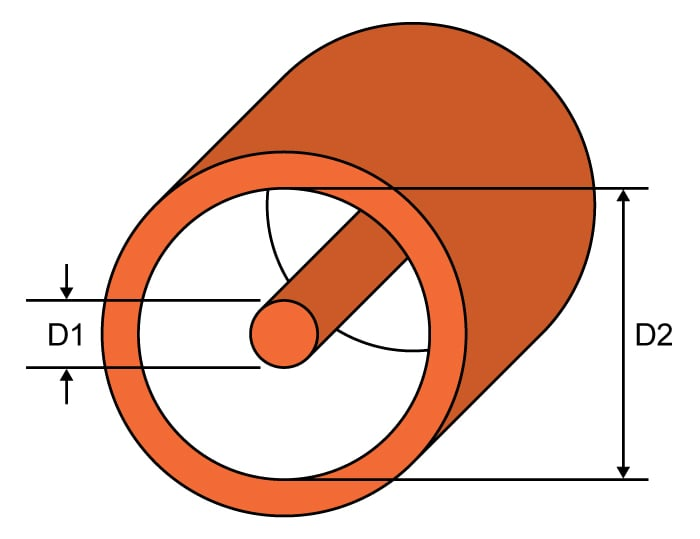# Coax Impedance Calculator

## A helpful tool for calculating the impedance of a coaxial cable.

Ohms
ns/in
nH/in
pF/in

### Overview

The coaxial cable, along with the balanced two-wire, is the most common type of transmission line used in RF communications. This calculator helps you calculate the characteristic impedance of a coaxial cable given its dimensions. This will also provide the time delay the cable provides for a signal and also the capacitance and inductance per unit length. You can choose the measurement unit for both the inner conductor and outer conductor. Note: The inner surface shield diameter is always greater than the inner conductor diameter.### Equations

$$Z_{0} = \frac{60}{\sqrt{\epsilon_{r}}} ln \frac{d_{2}}{d_{1}}$$

$$t_{d} = 84.72 \text{ x }10^{-3} \sqrt{\epsilon_{r}}$$

$$L = 5.08 ln (\frac{d_{2}}{d_{1}})$$

$$C = \frac{1.41 }{ ln (\frac{d_{2}}{d_{1}})}$$

Where:

$$Z_{0}$$ = characteristic impedance in ohms (Ω)

$$t_{d}$$ = time delay in nanoseconds per inch (ns/in)

$$L$$ = inductance per unit length in nanohenries per inch (nH/in)

$$C$$ = capacitance per unit length in picofarad per inch (pF/in)

$$d_{2}$$ = inner surface shield diameter

$$d_{1}$$ = inner conductor diameter

### Application

The coaxial cable is perhaps the most widely used type of transmission line. It consists of a solid center conductor surrounded by a dielectric material, usually a plastic insulator such as Teflon. An air or gas dielectric, in which the center conductor is held in place by periodic insulating spacers, can also be used. Over the insulator is a second conductor, a tubular braid or shield made of fine wires. An outer plastic sheath protects and insulates the braid.

The major benefit of coaxial cable is that it is completely shielded so that external noise has little or no effect on it. Coaxial cables are unbalanced lines; the current in the center conductor is referenced to the braid, which is connected to ground. Coaxial cables provide significant but not complete protection from noise pickup or crosstalk from inductive or capacitive coupling due to external signals. In contrast, unshielded lines may pick up signals and crosstalk and can even radiate energy, resulting in an undesirable loss of signal.

It is necessary to know the characteristic impedance of coaxial cables because there is a need to match the impedance of the cable to where it is attached to (whether a transmitter or a antenna) for maximum power transfer. Coaxial cables typically have a lower impedance compared to balanced lines and dipole antennas. In this case, a balun (balanced to unbalanced) transformer is often used to match them.